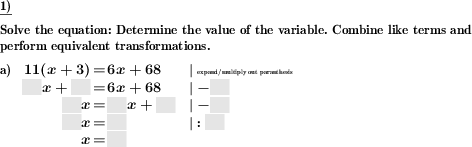Custom math worksheets at your fingertipsDetails for problem "Solve linear equation with distributive element"

Quickname: 3102

Junior High School, Middle School, High School.

Summary

Solve a linear equation step-by-step by performing equivalent transformations.

ExampleDescription

In a linear equation with one unknown variable, the value of the variable must be determined. The linear equation is formed by a sum of terms of the form ax and/or a on both sides of the equality sign. In addition, there is also a summand in the form of a distributive element a(x+b). By expanding the distributive element, combining like terms and performing equivalence transformation, the equation is to be brought into the form x=c, with c being a whole number. This term represents the solution of the equation. A corresponding note to use the technique of equivalent transformation can be given or omitted in the problem statement.

The number of terms to the left and right of the equality sign that appear together with the distributive element can be predetermined. If desired, only the variable x appears as an unknown in order not to confuse. The individual steps of the solution process can be selected to be

• not shown at all
• shown only in the solution or
• shown on the worksheet as well as on the solution sheet. On the worksheet, they will be presented as gap text.

The steps of equivalent transformations are shown in the order:

1. Multiply out/expand the distributive element
2. Summarize and rearrange, combine like terms
3. Move constant to the right side of the equality sign
4. Move variable elements to the left of the equality sign
5. Divide by the factor of the variable

The solution is always a whole number.

Download free printable worksheets for this math problem here. The worksheet contains the problems only, the solution sheet includes the answers. Just click on the respective link.

•Worksheet 1Solution sheet with answers
•Worksheet 2Solution sheet with answers
•Worksheet 3Solution sheet with answers

If you can not see the solution sheets for download, they may be filtered out by an ad blocker that you may have installed. If this is the case, please allow ads for this page and reload the page. The solution sheets will then reappear.

• Do these sample worksheets do not really fit?
• Do you need more math worksheets, with a different level of difficulty?
• Would you like to combine different problems on a worksheet and adjust them to your needs?
• As a teacher, you can put together your own worksheets using the automatically generated math problems provided.
With a free initial credit, you can start creating your own math worksheets in a few minutes.

You can try it for free! Register here, to create custom worksheets now!

Customization options for this problem

Parameter
Possible values
Number of problems
1, 2, 3, 4, 5, 6
Mention equivalent transformation
Yes, No
Show calculation steps
no, with solution, with problem&solution
Variable always x
Yes, No
Extra terms
1, 2, 3, 4, 5, 6
Distributive element always first
Yes, No

Similar problems

Remark
Description
use Gaussian Algorithm for linear equation system
A system of linear equations is to be solved with the Gaussian elimination method.
Simpler variant, without the distributive element
Solve a linear equation step-by-step by performing equivalent transformations.Deutsche Version dieser Aufgabe
These informational pages with samples describe math problems that can be combined on custom math worksheets with solutions for home and K-12 school use.
Deutsche Seiten
×